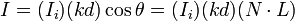# Lambert's law

Lambert's law : The law that the illumination of a surface by a light ray varies as the cosine of the angle of incidence between the normal to the surface and the incident ray. The law that the luminous intensity in a given direction radiated or reflected by a perfectly diffusing plane surface varies as the cosine of the angle between that direction and the normal to the surface.

## Lambert's cosine law

In optics - Lambert's cosine law : The mathematical statement that the radiance of certain idealized optical sources is directly proportional to the cosine of the angle - with respect to the direction of maximum radiance - from which the source is viewed. Lambert's cosine law may also apply to certain idealized diffuse reflectors.
An important consequence of Lambert's cosine law is that when an area element on the surface is viewed from any angle, it has the same apparent brightness. This is because although the emitted intensity from an area element is reduced by the cosine of the emission angle, the observed size (solid angle) of the area element is also reduced by that same amount, so that while the area element appears smaller, its brightness is the same. For example, in the visible spectrum, the Sun is almost a Lambertian radiator, and as a result the brightness of the Sun is almost the same everywhere on an image of the solar disk. Also, a perfect black body is a perfect Lambertian radiator.

## Lambertian reflectors

When an area element is radiating as a result of being illuminated by an external source, the flux (energy/time/area or photons/time/area) landing on that area element will be proportional to the cosine of the angle between the illuminating source and the normal. A Lambertian reflector will then reflect this light according to the same cosine law as a Lambertian emitter. This will mean that, although the apparent brightness of the surface will depend on the angle from the normal to the illuminating source, it will not depend on the angle from the normal to the observer. For example, if the moon were a Lambertian reflector, one would expect to see its reflected brightness appreciably diminish towards the outer edge, or limb. The fact that it does not illustrates that the moon is not a Lambertian reflector, and in fact tends to reflect more light into the oblique angles than a Lambertian reflector.

## Equations

Lambert cosine law formula represents as follows:$I = (I_i)( kd ) \cos \theta = (I_i) (kd)(N \cdot L)$
L unit light vector; N unit normal vector; I the intensity of the reflected light; Ii: the intensity of the incident light; kd diffuse reflectance